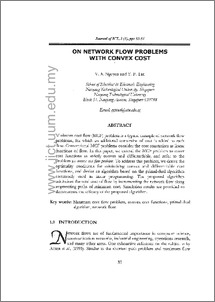UUM Repository | Universiti Utara Malaysian Institutional Repository
FAQs | Feedback | Search Tips | Sitemap

# On network flow problems with convex cost

Nguyen, V.A. and Tan, Y. P. (2004) On network flow problems with convex cost. Journal of ICT, 3 (1). pp. 33-51. ISSN 1675-414XPreview
PDF
Download (4MB) | Preview
Official URL: http://jict.uum.edu.my

## Abstract

Minimum cost flow (MCF) problem is a typical example of network flow problems, for which an additional constraint of cost is added to each flow. Conventional MCF problems consider the cost constraints as linear functions of flow. In this paper, we extend the MCF problem to cover cost functions as strictly convex and differentiable, and refer to the problem as convex cost flow problem. To address this problem, we derive the optimality conditions for minimising convex and differentiable cost functions, and devise an algorithm based on the primal-dual algorithm commonly used in linear programming. The proposed algorithm minimises the total cost of flow by incrementing the network flow along augmenting paths of minimum cost. Simulation results are provided to demonstrate the efficacy of the proposed algorithm.

Item Type: Article Minimum cost flow problem, convex cost functions, primal-dual, algorithm, network flow Q Science > QA Mathematics > QA75 Electronic computers. Computer science UNSPECIFIED Mrs. Norazmilah Yaakub 05 Sep 2010 04:57 05 Sep 2010 06:06 http://repo.uum.edu.my/id/eprint/1042

### Actions (login required)View Item